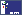# Berechnung des Seitenverhältnisses der Nationalfahne von Nepal

## Geometric / Algebraic Construction of the Flag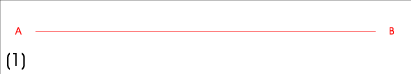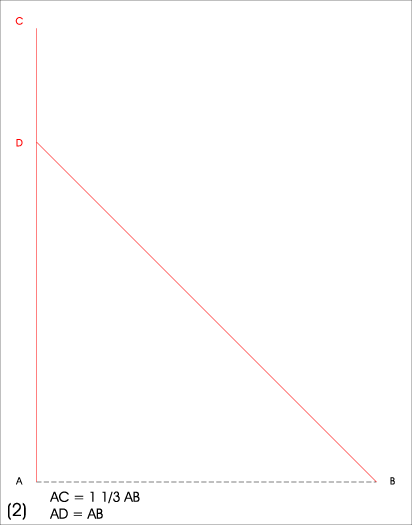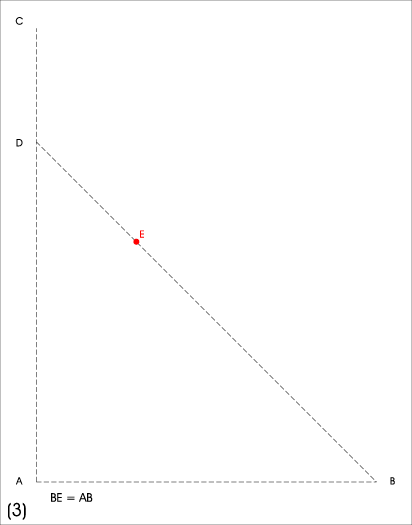Let A= (0,0), B= (3,0) => AB = 3 => C= (0,4), D= (0,3) => AD = 3, AC = 4 => BE = 3 E= (3-3/Sqrt(2) , 3/Sqrt(2))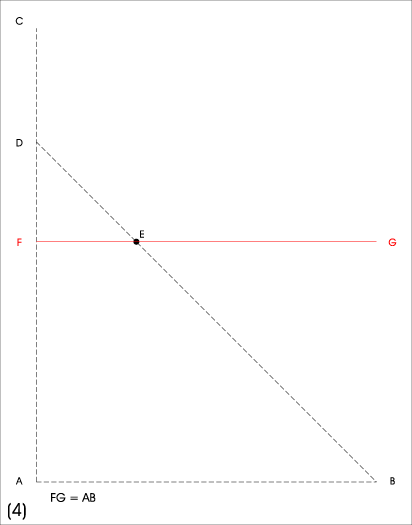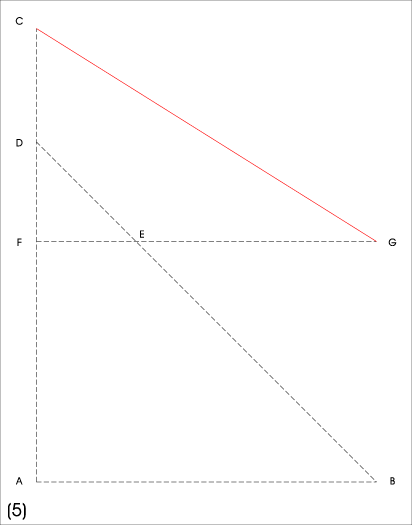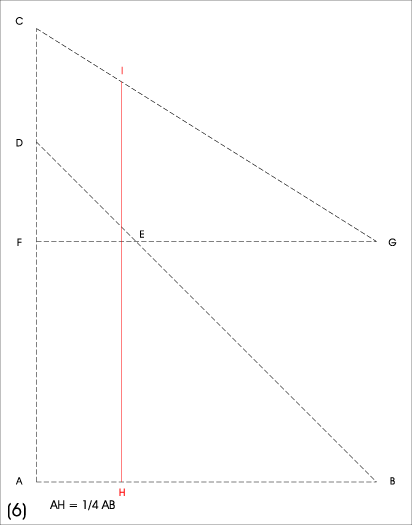=> FG = 3 F= (0, 3/Sqrt(2)) => AF = 3/Sqrt(2) , CF = 4- 3/Sqrt(2) G= (3, 3/Sqrt(2))) AH = 3/4 H= (3/4 , 0) I= (3/4, 3 + 3/4/Sqrt(2))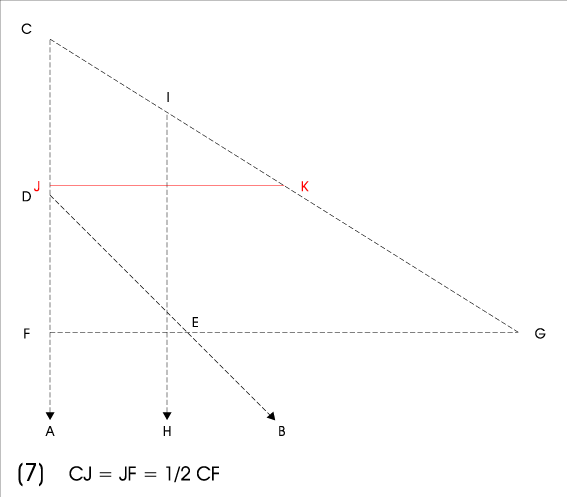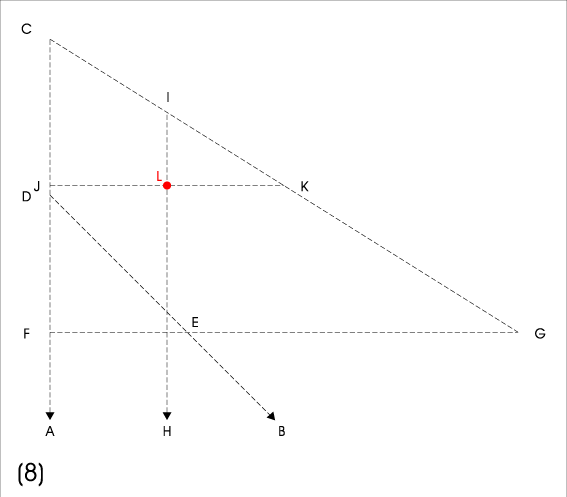J= (0, 2 + 3/2/Sqrt(2)) K= (3/2, 2 + 3/2/Sqrt(2)) L= (3/4, 2 + 3/2/Sqrt(2))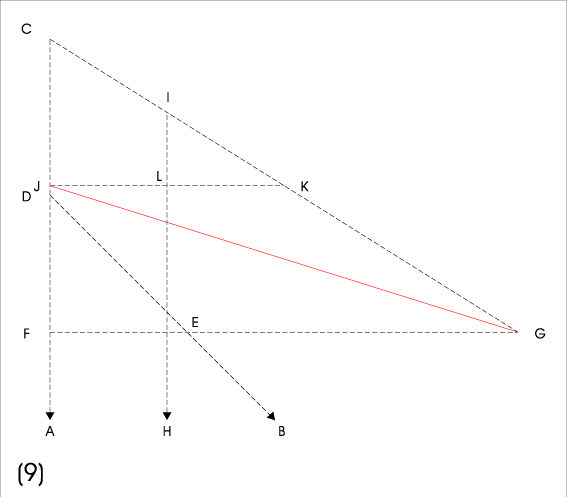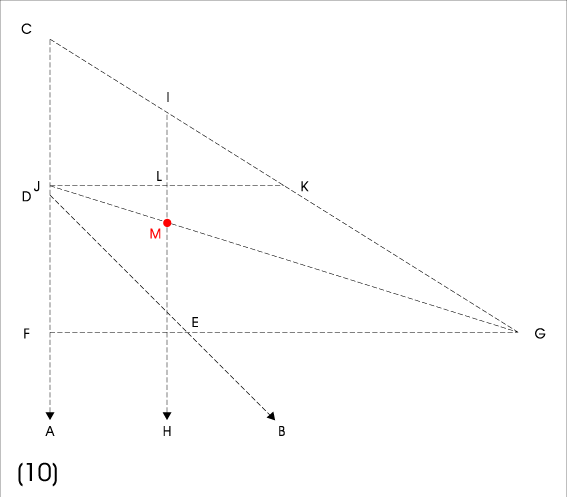M= (3/4 , 2 + 3/2/Sqrt(2) - 1/4*(4- 3/Sqrt(2))/2)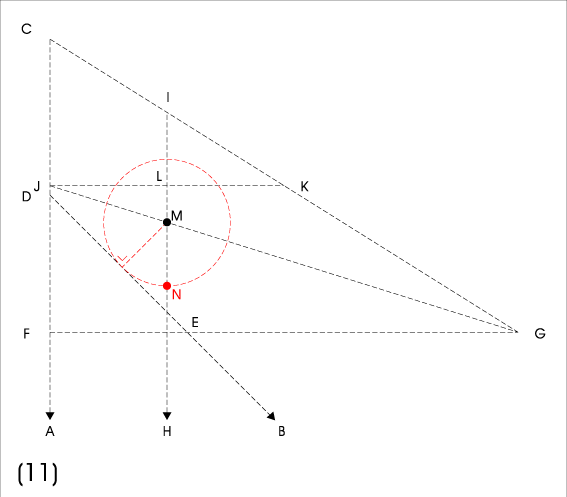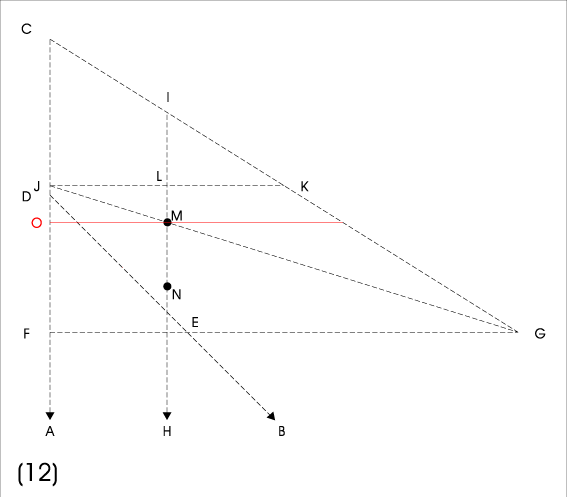x= point where IH cuts DB = ( 3/4 , 3-3/4) xM = -3/4 + 15/8/Sqrt(2) MN = xM/Sqrt(2) = 15/16-3/4/Sqrt(2) N = (3/4 , 9/16 + 21/8/Sqrt(2)) O = (0, 3/2 + 15/8/Sqrt(2)) LN= 23/16 - 9/8/Sqrt(2)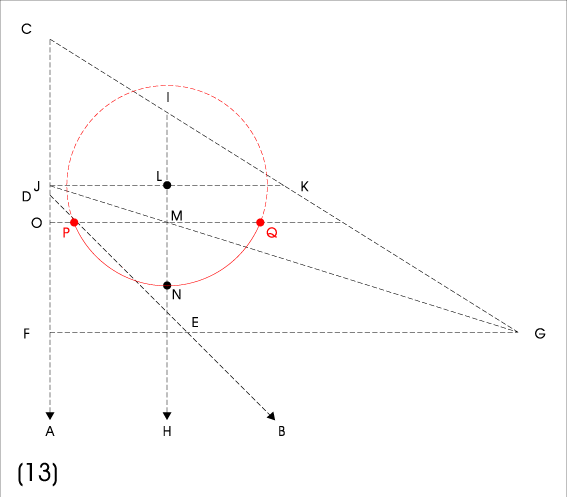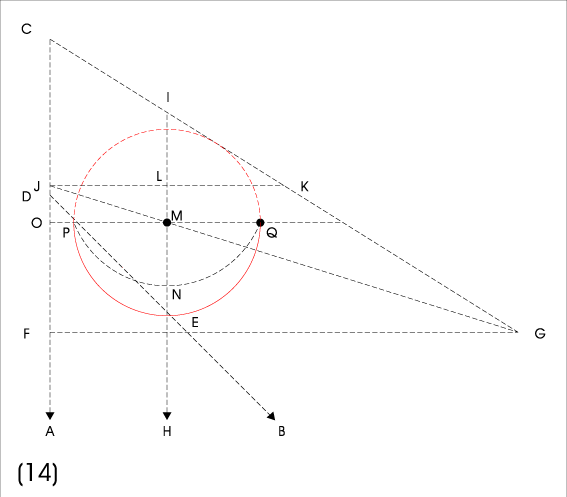circle around L with radius LN Q = (3/4 + Sqrt(609-366*Sqrt(2))/16 , 3/2 + 15/8/Sqrt(2)) P = (3/4 - Sqrt(609-366*Sqrt(2))/16 , 3/2 + 15/8/Sqrt(2))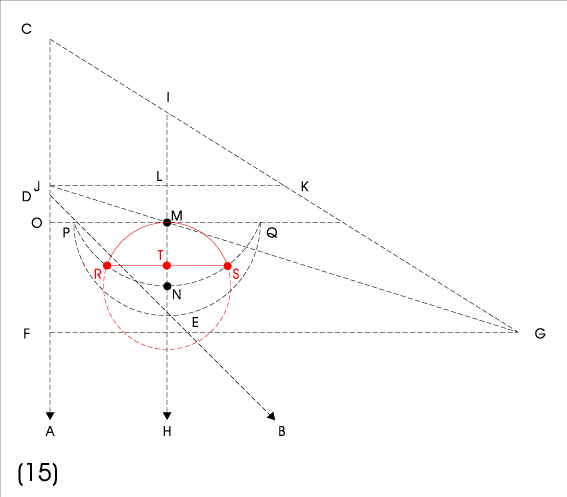circle around N with radius MN both circles cut each others at R and S at height h T = (3/4, h) TS = MN*Sqrt(1-(MN/2/LN)^2)

```consider the triangle (L,N,S) with lengths of sides  LN, LN, MN.

Bisecting it into two rectangle triangles

we get:  NS^2 + NT^2 = MN^2
and      NS^2 + LT^2 = LN^2

=>  MN^2-NT^2 = LN^2-LT^2
moreover we have LT+NT = LN , thus we can eliminate NT getting
MN^2-(LN-LT)^2 = LN^2-LT^2
or
MN^2-LN^2 +2*LT*LN = LN^2
or
LT =  (2*LN^2-MN^2)/(2*LN) = LN-MN^2/(2*LN)

now we can calculate h starting at the y-coordinate of L as

h = 2 + 3/2/Sqrt(2) - LT
= 2 + 3/2/Sqrt(2) -LN+MN^2/2/LN
= 9/16 + 21/8/Sqrt(2) + MN^2/LN/2
= 9/16 + 21/8/Sqrt(2) + (15/16-3/4/Sqrt(2))^2/(23/16 - 9/8/Sqrt(2))/2

= 9/16 +21/8/Sqrt(2) + (225/256+9/32-90/64/Sqrt(2))/(23/16 - 9/8/Sqrt(2))/2
= 9/16 +21/8/Sqrt(2) + (297/512-45/64/Sqrt(2))/(23/16 - 9/8/Sqrt(2))
= 9/16 +21/8/Sqrt(2) + (297/512-45/64/Sqrt(2))*(23/16 + 9/8/Sqrt(2))/(529/256-81/128)
= 9/16 +21/8/Sqrt(2) + (297/512-45/64/Sqrt(2))*(23/16 + 9/8/Sqrt(2))/(367/256)
= 9/16 +21/8/Sqrt(2) + (297/32-45/4/Sqrt(2))*(23 + 18/Sqrt(2))/367
= 9/16 +21/8/Sqrt(2) + (297*23/32-45*23/4/Sqrt(2)+297*18/32/Sqrt(2)-45/4*18/2)/367
= 9/16 +21/8/Sqrt(2) + (6831/32-8280/32/Sqrt(2)+5346/32/Sqrt(2)-810/8)/367
= 9/16 +21/8/Sqrt(2) + (3591/32-2934/32/Sqrt(2))/367

= 10197/367/32 + 27894/367/32/Sqrt(2)  ~ 2.54777218616

and using again  LT+NT = LN  we get

TN = NT = MN^2/(2*LN) = (3591-2934/Sqrt(2))/32/367
TS = MN*Sqrt(1-(MN/2/LN)^2)
= 3*Sqrt(2467 - 1476*Sqrt(2))*(-5+2*Sqrt(2)) / 32 / (-23+9*Sqrt(2))
= (237-3*Sqrt(2))*Sqrt(2467-1476*Sqrt(2))/11744   ~ 0.38615562772035130798
```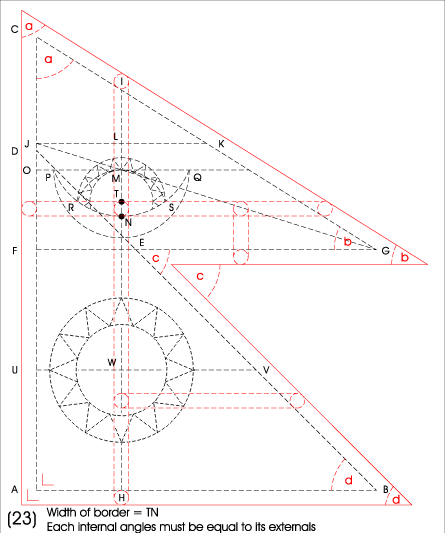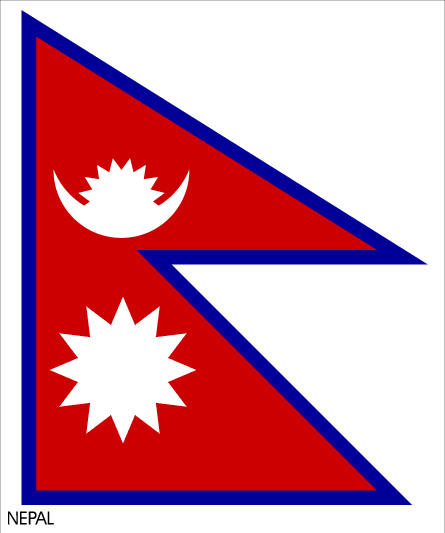AU = AF/2 W= (3/4, 3/2/Sqrt(2)) m := TN = NT = MN^2/(2*LN) ~ 0.1291168855584698 lower left corner angel at A = 90 ° = Pi/2 lower right corner angel d = 45 ° = Pi/4 middle inner corner angel c = -45 ° = -Pi/4 Pi/2 - upper left corner angle a = right outer corner angle b =: w National Flag of Nepal used since at least December 1962
```t := tan(w) = 4/3 - 1/Sqrt(2)
w = arctan(4/3 - 1/Sqrt(2))   ~ 0.1780882838*Pi

length of bottom line:  m+3+m+m*Sqrt(2)  ~ 3.44083262177

tan(w) = x/(m+m/sin(w)) = x/m/(1+Sqrt(1+tan^2(w))/tan(w))
x = m*(tan(w)+Sqrt(1+tan^2(w)))

x= m*(1+1/sin(w))*(4/3-1/Sqrt(2)) = m*(tan(w)+1/cos(w))
length of left vertical line:  m+4+x  ~ 4.3623180847787892670

z = m/tan(w/2) = m*(1+cos(w))/sin(w) = m*(1/sin(w)+1/tan(w))
= m*(Sqrt(1+tan^2(w))+1)/tan(w)
length of horicontal middle peak:  m+3+z   ~ 3.5785734947467747880

ratio resp. Verhältnis = (m+4+x)/(m+3+z)   ~ 1.219010337829452

=  (3591-2934/Sqrt(2))/367/32+4+(3591-2934/Sqrt(2))/367/32*(t+Sqrt(1+t^2))/
((3591-2934/Sqrt(2))/367/32+3+(3591-2934/Sqrt(2))/367/32*(1+Sqrt(1+t^2))/t)

=  (3591-2934/Sqrt(2))+4*367*32+(3591-2934/Sqrt(2))*(t+Sqrt(1+t^2))/
((3591-2934/Sqrt(2))+3*367*32+(3591-2934/Sqrt(2))*(1+Sqrt(1+t^2))/t)

1+t^2 = 1+(4/3-1/Sqrt(2))^2 = 1+ 16/9+1/2-8/3/Sqrt(2) = 32/18+27/18-8/3/Sqrt(2)
Sqrt(1+t^2) = Sqrt(59/18-8/3/Sqrt(2)) = Sqrt(59/2-24/Sqrt(2))/3

(m+4+x)= (Sqrt(118-48*Sqrt(2))*(1197-489*Sqrt(2))-10437*Sqrt(2)+113644)/23488

(m+3+z)= (Sqrt(118-48*Sqrt(2))*(19926-963*Sqrt(2))-73260*Sqrt(2)+1905414)/540224

(m+4+x)/(m+3+z)= 23*(Sqrt(118-48*Sqrt(2))*(1197-489*Sqrt(2))-10437*Sqrt(2)+113644)*(Sqrt(118-48*Sqrt(2))*(19926-963*Sqrt(2))+73260*Sqrt(2)-1905414)/(255505506840*Sqrt(2)-3590582103648)
= (Sqrt(118-48*Sqrt(2))*(16146755136*Sqrt(2)-1560707136)+591939501840*Sqrt(2)-4924217880984)/(255505506840*Sqrt(2)-3590582103648)
= (Sqrt(118-48*Sqrt(2))*(702032832*Sqrt(2)-67856832)+25736500080*Sqrt(2)-214096429608)/(11108935080*Sqrt(2)-156112265376)
= (Sqrt(118-48*Sqrt(2))*(29251368*Sqrt(2)-2827368)+1072354170*Sqrt(2)-8920684567)/(462872295*Sqrt(2)-6504677724)
= (6136891429688-306253616715*Sqrt(2)-Sqrt(118-48*Sqrt(2))*(934861968+20332617192*Sqrt(2)))/4506606337686

= 1.219010337829452184570024869930988566...

is the value of the sides-ratio of the circumscribing rectangle of the flag.
```
```   r - s*Sqrt(2) - Sqrt(118-48*Sqrt(2)) * (u+v*Sqrt(2))
= ------------------------------------------------------
w

with

r = 6136891429688
s = 306253616715
u = 934861968
v = 20332617192
w = 4506606337686

corner coordinates of the flag (A:B:C = 0:3:4) :

at lower left (near A): (-m,-m)
at upper left (near C): (-m,4+x)
at lower right (near B): (3+m+m*Sqrt(2),-m)
at middle inner (near E): (3-3/Sqrt(2)+m+m*Sqrt(2),3/Sqrt(2)-m)
at middle outer (near G): (3+z,3/Sqrt(2)-m)

with m = 9*(399-163*Sqrt(2))/11744
x = m*(8-3*Sqrt(2)+Sqrt(118-48*Sqrt(2)))/6
z = m*(3+Sqrt(59/2-24/Sqrt(2)))/(4-3/Sqrt(2))

the area of the flag is ~ 9.05889579867594   or
A = (43590392214+7537121109*Sqrt(2)+(1176214419-509719833*Sqrt(2))*Sqrt(118-48*Sqrt(2)))/6344390656
```

Remark: This page sprang of a private working paper which was not intended for publication. Sorry, for the plain layout.

First time calculated at 2012-04-13 18:38 UTC+2

## References

Constitution of The Kingdom of Nepal, Article 5, Shedule 1 translated in english.
All geometric sheets for the Nepalesian Flag at FOTW Thanks to Sammy Kanadi.
Further background information on Nepal's Flag Thanks to